Courses

# Test: Data Interpretation - 2 (2014-2011)

## 15 Questions MCQ Test UPSC Topic Wise Previous Year Questions | Test: Data Interpretation - 2 (2014-2011)

Description
This mock test of Test: Data Interpretation - 2 (2014-2011) for UPSC helps you for every UPSC entrance exam. This contains 15 Multiple Choice Questions for UPSC Test: Data Interpretation - 2 (2014-2011) (mcq) to study with solutions a complete question bank. The solved questions answers in this Test: Data Interpretation - 2 (2014-2011) quiz give you a good mix of easy questions and tough questions. UPSC students definitely take this Test: Data Interpretation - 2 (2014-2011) exercise for a better result in the exam. You can find other Test: Data Interpretation - 2 (2014-2011) extra questions, long questions & short questions for UPSC on EduRev as well by searching above.
QUESTION: 1

### The following graph shows the average profit of two fruit-sellers A and B in thousands (₹) per year from the year 1995 to 2000. Consider the graph and answer the 4 (four) items that follow :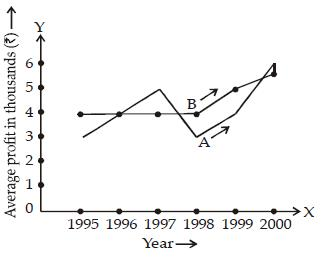Q. How much more average profit did A make in the year 2000 than in the year 1999? 

Solution:

In 2000, Profit of A = 6000
In 1999, profit of A = 4000
∴ Difference = ₹ 2000

QUESTION: 2

Solution:
QUESTION: 3

### Study the two figures given below and answer the questions that follow: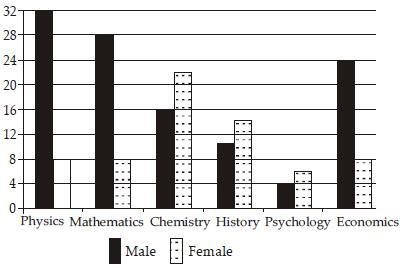Fig 1: Number of Professors in selected disciplines in a University by sex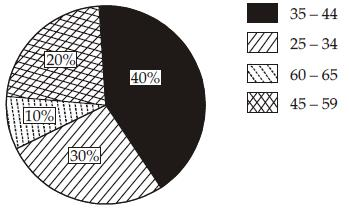Fig. 2 : Age of Physics Professors Q. How many Physics professors belong to the age group 35 – 44? 

Solution:

Number of professors in physics = 40 Percentage of professors in age group 35 – 44 = 40%
∴ Physics professors belong to age group
35 – 44 = 40% of 40
= 16

QUESTION: 4

Study the two figures given below and answer the questions that follow:Fig 1: Number of Professors in selected disciplines in a University by sexFig. 2 : Age of Physics Professors

Q. Which one of the following disciplines has the highest ratio of males to females?



Solution:

By looking to graph I. We can deduce that physics discipline has the highest ratio of males to females.

QUESTION: 5

Study the two figures given below and answer the questions that follow:Fig 1: Number of Professors in selected disciplines in a University by sexFig. 2 : Age of Physics Professors

Q. What percentage of all Psychology professors are females?



Solution:

No. of females psychology professor = 6 No. of males psychology professor = 4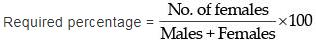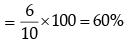QUESTION: 6

Study the two figures given below and answer the questions that follow:Fig 1: Number of Professors in selected disciplines in a University by sexFig. 2 : Age of Physics Professors

Q. If the number of female Physics professors in the age group 25 – 34 equals 25% of all the Physics professors in that age group, then what is the number of male Physics professors in the age group 25 – 34?



Solution:

Number of all physics professors in age group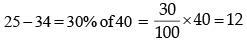Number of female physics professors in the age group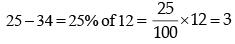∴ Number of male physics professors in the age group
25 – 34 = 12 – 3 = 9

QUESTION: 7

Study the two figures given below and answer the questions that follow:Fig 1: Number of Professors in selected disciplines in a University by sexFig. 2 : Age of Physics Professors

Q. If the Psychology professors in the University constitute 2% of all the professors in the University, then what is the number of professors in the University?



Solution:

Let the number of professors in the university = x
According to question
2% of x = 10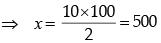QUESTION: 8

Consider the following diagrams:
x men, working at constant speed, do a certain job in y days. Which one of these diagrams shows the relation between x and y?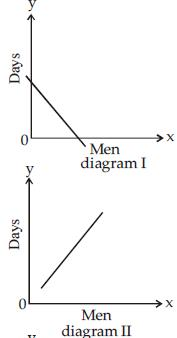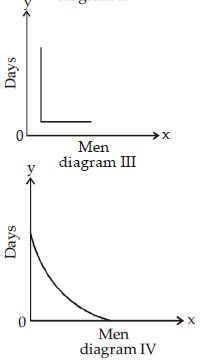Solution:

Time taken to finish a work is inversely proportional to the number of persons working at it.
∴ More persons then less is the time taken to finish a job. Hence, diagram IV represents the relation between x and y.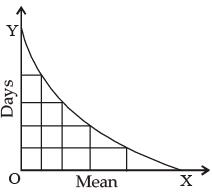QUESTION: 9

Consider the following information regarding the performance of a class of 1000 students in four different tests: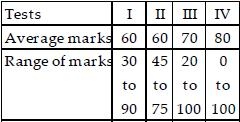If a student scores 74 marks in each of the four tests, in which one of the following tests is her performance the best comparatively?



Solution: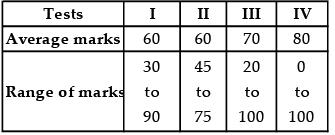90 – 30 = 75, 75 – 45 = 30
100 – 20 = 80 110 – 0 = 110
If a student scores 74 marks in each of the four tests, his performance would be the best comparatively in test II because there would be less numbers of the students in this range in test II only.

QUESTION: 10

Consider the following distance - time graph. The graph shows three athletes A, B and C running side by side for a 30 km race.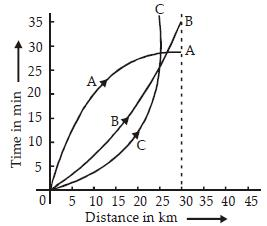With reference to the above graph, consider the following statements :
1. The race was won by A.
2. B was ahead mark of A up to 25 km mark
3. C ran very slowly from the beginning.
Which of the statements given above is/are correct?



Solution:

Since x axis shows the distance and y axis shows the time, hence lower graph will show faster athlete and higher graph will show slower athlete. Height of A's graph is the lowest, hence, the race was won by A. Height of B's graph is lower than A's graph up to 25 km, hence B was running faster than A up to 25 km. The height of C's graph is the lowest from the beginning, hence he run very fastly from the beginning, Hence statement 3 is not correct.

QUESTION: 11

Read the passage given below, study the graph that follows and answer the three items given below the figure.

During a party, a person was exposed to contaminated water. A few days later, he developed fever and loose motions. He suffered for some days before going to a doctor for treatment. On starting the treatment, he soon became better and recovered completely a few days later. The following graph shows different phases of the person's disease condition as regions A, B, C, D and E of the curve.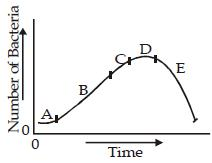Q. Which region/regions of the curve correspond/corresponds to incubation phase of the infection?

[2011

Solution:

Incubation phase means the time between somebody being infected with a diseases in graph part A shows that phase.

QUESTION: 12

Read the passage given below, study the graph that follows and answer the three items given below the figure.

During a party, a person was exposed to contaminated water. A few days later, he developed fever and loose motions. He suffered for some days before going to a doctor for treatment. On starting the treatment, he soon became better and recovered completely a few days later. The following graph shows different phases of the person's disease condition as regions A, B, C, D and E of the curve.Q. Which region of the curve indicates that the person began showing the symptoms of infection?

[2011

Solution:

Region B clearly shows the symptoms of infection.

QUESTION: 13

Read the passage given below, study the graph that follows and answer the three items given below the figure.

During a party, a person was exposed to contaminated water. A few days later, he developed fever and loose motions. He suffered for some days before going to a doctor for treatment. On starting the treatment, he soon became better and recovered completely a few days later. The following graph shows different phases of the person's disease condition as regions A, B, C, D and E of the curve.Q. Which region of the curve indicates that the treatment yielded effective relief?

[2011

Solution:

Though relief began from region C but only region E indicates effective relief.

QUESTION: 14

Consider the following Velocity – Time graph. It shows two trains starting simultaneously on parallel tracks.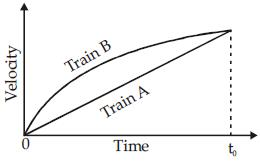With reference to the above graph, which one of the following statements is not correct?



Solution:

Distance = time x speed, here train B is faster than train A, hence Train B will cover more distance than train A.

QUESTION: 15

The following pie charts show the break up of disease categories recorded in the patients from two towns, Town A and Town B. Pie charts plot the disease categories as percentage of the total number of patients. Based on these answer the two items that follow the charts.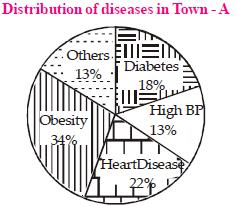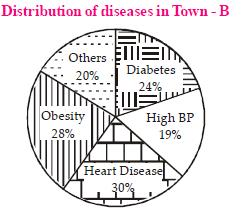Q. What can we say about persons with more than one disease from these graphs?



Solution:

The sum of percentage of patients in town A is 100, hence there is no any patient with more than one disease.